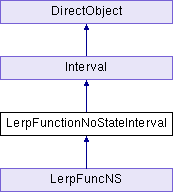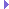LerpFunctionNoStateInterval Class Reference
Inheritance diagram for LerpFunctionNoStateInterval:## Public Member Functions

def __init__ (self, function, duration=0.0, fromData=0, toData=1, blendType='noBlend', extraArgs=[], name=None)

def privStep (self, t)
Evaluate function apply(self.function, [data] + self.extraArgs) self.state = CInterval.SStarted self.currT = t. More...Public Member Functions inherited from Interval
def __init__ (self, name, duration, openEnded=1)

def __repr__ (self, indent=0)

def clearToInitial (self)

def finish (self)

def getDoneEvent (self)

def getDuration (self)

def getLoop (self)

def getName (self)

def getOpenEnded (self)

def getPlayRate (self)

def getState (self)

def getT (self)

def intervalDone (self)

def isPaused (self)

def isPlaying (self)

def isStopped (self)

def loop (self, startT=0.0, endT=-1.0, playRate=1.0)

def pause (self)

def popupControls (self, tl=None)

def privDoEvent (self, t, event)

def privFinalize (self)

def privInitialize (self, t)

def privInstant (self)

def privInterrupt (self)

def privPostEvent (self)

def privReverseFinalize (self)

def privReverseInitialize (self, t)

def privReverseInstant (self)

def resume (self, startT=None)

def resumeUntil (self, endT)

def setDoneEvent (self, event)

def setLoop (self, loop=1)

def setPlayRate (self, playRate)

def setT (self, t)

def setupPlay (self, startT, endT, playRate, doLoop)

def setupResume (self)

def start (self, startT=0.0, endT=-1.0, playRate=1.0)

def stepPlay (self)Public Member Functions inherited from DirectObject
def __init__ (self)

def accept (self, event, method, extraArgs=[])

def acceptOnce (self, event, method, extraArgs=[])

def addTask (self, *args, **kwargs)

def detectLeaks (self)

def doMethodLater (self, *args, **kwargs)

def getAllAccepting (self)

def ignore (self, event)

def ignoreAll (self)

def isAccepting (self, event)

def isIgnoring (self, event)

def removeAllTasks (self)

def removeTask (self, taskOrName)

## Public Attributes

blendType

currT

duration

extraArgs

fromData

function

state

toDataPublic Attributes inherited from Interval
currT

doneEvent

duration

es

name

openEnded

pname

pstats

setTHooks

state

## Static Public Attributes

int lerpFunctionIntervalNum = 1

notify = directNotify.newCategory('LerpFunctionNoStateInterval')Static Public Attributes inherited from Interval
notify = directNotify.newCategory("Interval")

int playbackCounter = 0Static Public Attributes inherited from DirectObject
def accept_once = acceptOnce

def add_task = addTask

def detect_leaks = detectLeaks

def do_method_later = doMethodLater

def get_all_accepting = getAllAccepting

def ignore_all = ignoreAll

def is_accepting = isAccepting

def is_ignoring = isIgnoring

def remove_all_tasks = removeAllTasks

def remove_task = removeTask

## Additional Inherited MembersProperties inherited from Interval
done_event = property(getDoneEvent, setDoneEvent)

open_ended = property(getOpenEnded)

play_rate = property(getPlayRate, setPlayRate)

stopped = property(isStopped)

t = property(getT, setT)

## Detailed Description

```Class used to execute a function over time.  Function can access fromData
and toData to perform blend.  If fromData and toData not specified, will
execute the given function passing in values ranging from 0 to 1

This is different from a standard LerpFunction, in that it assumes
the function is not modifying any state that needs to be kept; so
that it will only call the function while the lerp is actually
running, and will not be guaranteed to call the function with its
final value of the lerp.  In particular, if the lerp interval
happens to get skipped over completely, it will not bother to call
the function at all.
```

## ◆ __init__()

 def __init__ ( self, function, duration = `0.0`, fromData = `0`, toData = `1`, blendType = `'noBlend'`, extraArgs = `[]`, name = `None` )
```__init__(function, duration, fromData, toData, name)
```

## ◆ privStep()

 def privStep ( self, t )

Evaluate function apply(self.function, [data] + self.extraArgs) self.state = CInterval.SStarted self.currT = t.

Reimplemented from Interval.

## ◆ blendType

 blendType

 currT

## ◆ duration

 duration

## ◆ extraArgs

 extraArgs

## ◆ fromData

 fromData

## ◆ function

 function

## ◆ lerpFunctionIntervalNum

 int lerpFunctionIntervalNum = 1
static

## ◆ notify

 notify = directNotify.newCategory('LerpFunctionNoStateInterval')
static

 state

 toData